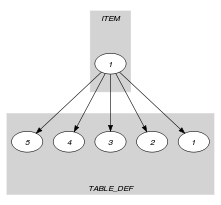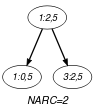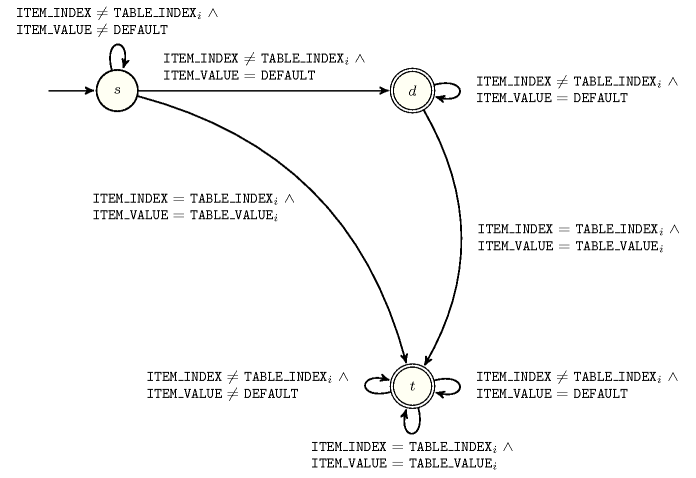## 5.144. element_sparse

Origin

CHIP

Constraint

$\mathrm{𝚎𝚕𝚎𝚖𝚎𝚗𝚝}_\mathrm{𝚜𝚙𝚊𝚛𝚜𝚎}\left(\mathrm{𝙸𝚃𝙴𝙼},\mathrm{𝚃𝙰𝙱𝙻𝙴},\mathrm{𝙳𝙴𝙵𝙰𝚄𝙻𝚃}\right)$

Usual name

$\mathrm{𝚎𝚕𝚎𝚖𝚎𝚗𝚝}$

Arguments
 $\mathrm{𝙸𝚃𝙴𝙼}$ $\mathrm{𝚌𝚘𝚕𝚕𝚎𝚌𝚝𝚒𝚘𝚗}\left(\mathrm{𝚒𝚗𝚍𝚎𝚡}-\mathrm{𝚍𝚟𝚊𝚛},\mathrm{𝚟𝚊𝚕𝚞𝚎}-\mathrm{𝚍𝚟𝚊𝚛}\right)$ $\mathrm{𝚃𝙰𝙱𝙻𝙴}$ $\mathrm{𝚌𝚘𝚕𝚕𝚎𝚌𝚝𝚒𝚘𝚗}\left(\mathrm{𝚒𝚗𝚍𝚎𝚡}-\mathrm{𝚒𝚗𝚝},\mathrm{𝚟𝚊𝚕𝚞𝚎}-\mathrm{𝚒𝚗𝚝}\right)$ $\mathrm{𝙳𝙴𝙵𝙰𝚄𝙻𝚃}$ $\mathrm{𝚒𝚗𝚝}$
Restrictions
 $\mathrm{𝚛𝚎𝚚𝚞𝚒𝚛𝚎𝚍}$$\left(\mathrm{𝙸𝚃𝙴𝙼},\left[\mathrm{𝚒𝚗𝚍𝚎𝚡},\mathrm{𝚟𝚊𝚕𝚞𝚎}\right]\right)$ $\mathrm{𝙸𝚃𝙴𝙼}.\mathrm{𝚒𝚗𝚍𝚎𝚡}\ge 1$ $|\mathrm{𝙸𝚃𝙴𝙼}|=1$ $|\mathrm{𝚃𝙰𝙱𝙻𝙴}|>0$ $\mathrm{𝚛𝚎𝚚𝚞𝚒𝚛𝚎𝚍}$$\left(\mathrm{𝚃𝙰𝙱𝙻𝙴},\left[\mathrm{𝚒𝚗𝚍𝚎𝚡},\mathrm{𝚟𝚊𝚕𝚞𝚎}\right]\right)$ $\mathrm{𝚃𝙰𝙱𝙻𝙴}.\mathrm{𝚒𝚗𝚍𝚎𝚡}\ge 1$ $\mathrm{𝚍𝚒𝚜𝚝𝚒𝚗𝚌𝚝}$$\left(\mathrm{𝚃𝙰𝙱𝙻𝙴},\mathrm{𝚒𝚗𝚍𝚎𝚡}\right)$
Purpose

$\mathrm{𝙸𝚃𝙴𝙼}\left[1\right].\mathrm{𝚟𝚊𝚕𝚞𝚎}$ is equal to one of the entries of the table $\mathrm{𝚃𝙰𝙱𝙻𝙴}$ or to the default value $\mathrm{𝙳𝙴𝙵𝙰𝚄𝙻𝚃}$ if the entry $\mathrm{𝙸𝚃𝙴𝙼}\left[1\right].\mathrm{𝚒𝚗𝚍𝚎𝚡}$ does not exist in $\mathrm{𝚃𝙰𝙱𝙻𝙴}$.

Example
$\left(\begin{array}{c}〈\mathrm{𝚒𝚗𝚍𝚎𝚡}-2\mathrm{𝚟𝚊𝚕𝚞𝚎}-5〉,\hfill \\ 〈\begin{array}{cc}\mathrm{𝚒𝚗𝚍𝚎𝚡}-1\hfill & \mathrm{𝚟𝚊𝚕𝚞𝚎}-6,\hfill \\ \mathrm{𝚒𝚗𝚍𝚎𝚡}-2\hfill & \mathrm{𝚟𝚊𝚕𝚞𝚎}-5,\hfill \\ \mathrm{𝚒𝚗𝚍𝚎𝚡}-4\hfill & \mathrm{𝚟𝚊𝚕𝚞𝚎}-2,\hfill \\ \mathrm{𝚒𝚗𝚍𝚎𝚡}-8\hfill & \mathrm{𝚟𝚊𝚕𝚞𝚎}-9\hfill \end{array}〉,5\hfill \end{array}\right)$

The $\mathrm{𝚎𝚕𝚎𝚖𝚎𝚗𝚝}_\mathrm{𝚜𝚙𝚊𝚛𝚜𝚎}$ constraint holds since its first argument $\mathrm{𝙸𝚃𝙴𝙼}$ corresponds to the second item of the $\mathrm{𝚃𝙰𝙱𝙻𝙴}$ collection.

Typical
 $|\mathrm{𝚃𝙰𝙱𝙻𝙴}|>1$ $\mathrm{𝚛𝚊𝚗𝚐𝚎}$$\left(\mathrm{𝚃𝙰𝙱𝙻𝙴}.\mathrm{𝚟𝚊𝚕𝚞𝚎}\right)>1$
Symmetries
• Items of $\mathrm{𝚃𝙰𝙱𝙻𝙴}$ are permutable.

• All occurrences of two distinct values in $\mathrm{𝙸𝚃𝙴𝙼}.\mathrm{𝚟𝚊𝚕𝚞𝚎}$, $\mathrm{𝚃𝙰𝙱𝙻𝙴}.\mathrm{𝚟𝚊𝚕𝚞𝚎}$ or $\mathrm{𝙳𝙴𝙵𝙰𝚄𝙻𝚃}$ can be swapped; all occurrences of a value in $\mathrm{𝙸𝚃𝙴𝙼}.\mathrm{𝚟𝚊𝚕𝚞𝚎}$, $\mathrm{𝚃𝙰𝙱𝙻𝙴}.\mathrm{𝚟𝚊𝚕𝚞𝚎}$ or $\mathrm{𝙳𝙴𝙵𝙰𝚄𝙻𝚃}$ can be renamed to any unused value.

Usage

A sometimes more compact form of the $\mathrm{𝚎𝚕𝚎𝚖𝚎𝚗𝚝}$ constraint: we are not obliged to specify explicitly the table entries that correspond to the specified default value. This can sometimes reduce drastically memory utilisation.

Remark

The original constraint of CHIP had an additional parameter $\mathrm{𝚂𝙸𝚉𝙴}$ giving the maximum value of $\mathrm{𝙸𝚃𝙴𝙼}.\mathrm{𝚒𝚗𝚍𝚎𝚡}$.

Reformulation

Let $𝙸$ and $𝚅$ respectively denote $\mathrm{𝙸𝚃𝙴𝙼}\left[1\right].\mathrm{𝚒𝚗𝚍𝚎𝚡}$ and $\mathrm{𝙸𝚃𝙴𝙼}\left[1\right].\mathrm{𝚟𝚊𝚕𝚞𝚎}$. The $\mathrm{𝚎𝚕𝚎𝚖𝚎𝚗𝚝}_\mathrm{𝚜𝚙𝚊𝚛𝚜𝚎}$$\left(\mathrm{𝙸𝚃𝙴𝙼},\mathrm{𝚃𝙰𝙱𝙻𝙴},\mathrm{𝙳𝙴𝙵𝙰𝚄𝙻𝚃}\right)$ constraint can be expressed in term of a reified constraint of the form:

$\left(\left(𝙸=\mathrm{𝚃𝙰𝙱𝙻𝙴}\left[1\right].\mathrm{𝚒𝚗𝚍𝚎𝚡}\wedge 𝚅=\mathrm{𝚃𝙰𝙱𝙻𝙴}\left[1\right].\mathrm{𝚟𝚊𝚕𝚞𝚎}\right)\vee$

$\left(𝙸=\mathrm{𝚃𝙰𝙱𝙻𝙴}\left[2\right].\mathrm{𝚒𝚗𝚍𝚎𝚡}\wedge 𝚅=\mathrm{𝚃𝙰𝙱𝙻𝙴}\left[2\right].\mathrm{𝚟𝚊𝚕𝚞𝚎}\right)\vee$

$\cdots$

$\left(𝙸=\mathrm{𝚃𝙰𝙱𝙻𝙴}\left[|\mathrm{𝚃𝙰𝙱𝙻𝙴}|\right].\mathrm{𝚒𝚗𝚍𝚎𝚡}\wedge 𝚅=\mathrm{𝚃𝙰𝙱𝙻𝙴}\left[\mathrm{𝚃𝙰𝙱𝙻𝙴}|\right].\mathrm{𝚟𝚊𝚕𝚞𝚎}\right)\right)\vee$

$\left(\left(𝙸\ne \mathrm{𝚃𝙰𝙱𝙻𝙴}\left[1\right].\mathrm{𝚒𝚗𝚍𝚎𝚡}\right)\wedge$

$\left(𝙸\ne \mathrm{𝚃𝙰𝙱𝙻𝙴}\left[2\right].\mathrm{𝚒𝚗𝚍𝚎𝚡}\right)\wedge$

$\cdots$

$\left(𝙸\ne \mathrm{𝚃𝙰𝙱𝙻𝙴}\left[|\mathrm{𝚃𝙰𝙱𝙻𝙴}|\right].\mathrm{𝚒𝚗𝚍𝚎𝚡}\right)\wedge$

$\left(𝚅=\mathrm{𝙳𝙴𝙵𝙰𝚄𝙻𝚃}\right)\right)$.

Keywords
Derived Collections
 $\mathrm{𝚌𝚘𝚕}\left(\begin{array}{c}\mathrm{𝙳𝙴𝙵}-\mathrm{𝚌𝚘𝚕𝚕𝚎𝚌𝚝𝚒𝚘𝚗}\left(\mathrm{𝚒𝚗𝚍𝚎𝚡}-\mathrm{𝚒𝚗𝚝},\mathrm{𝚟𝚊𝚕𝚞𝚎}-\mathrm{𝚒𝚗𝚝}\right),\hfill \\ \mathrm{𝚒𝚝𝚎𝚖}\left(\mathrm{𝚒𝚗𝚍𝚎𝚡}-0,\mathrm{𝚟𝚊𝚕𝚞𝚎}-\mathrm{𝙳𝙴𝙵𝙰𝚄𝙻𝚃}\right)\right]\hfill \end{array}\right)$ $\mathrm{𝚌𝚘𝚕}\left(\begin{array}{c}\mathrm{𝚃𝙰𝙱𝙻𝙴}_\mathrm{𝙳𝙴𝙵}-\mathrm{𝚌𝚘𝚕𝚕𝚎𝚌𝚝𝚒𝚘𝚗}\left(\mathrm{𝚒𝚗𝚍𝚎𝚡}-\mathrm{𝚍𝚟𝚊𝚛},\mathrm{𝚟𝚊𝚕𝚞𝚎}-\mathrm{𝚍𝚟𝚊𝚛}\right),\hfill \\ \left[\begin{array}{c}\mathrm{𝚒𝚝𝚎𝚖}\left(\mathrm{𝚒𝚗𝚍𝚎𝚡}-\mathrm{𝚃𝙰𝙱𝙻𝙴}.\mathrm{𝚒𝚗𝚍𝚎𝚡},\mathrm{𝚟𝚊𝚕𝚞𝚎}-\mathrm{𝚃𝙰𝙱𝙻𝙴}.\mathrm{𝚟𝚊𝚕𝚞𝚎}\right),\hfill \\ \mathrm{𝚒𝚝𝚎𝚖}\left(\mathrm{𝚒𝚗𝚍𝚎𝚡}-\mathrm{𝙳𝙴𝙵}.\mathrm{𝚒𝚗𝚍𝚎𝚡},\mathrm{𝚟𝚊𝚕𝚞𝚎}-\mathrm{𝙳𝙴𝙵}.\mathrm{𝚟𝚊𝚕𝚞𝚎}\right)\hfill \end{array}\right]\hfill \end{array}\right)$
Arc input(s)

$\mathrm{𝙸𝚃𝙴𝙼}$ $\mathrm{𝚃𝙰𝙱𝙻𝙴}_\mathrm{𝙳𝙴𝙵}$

Arc generator
$\mathrm{𝑃𝑅𝑂𝐷𝑈𝐶𝑇}$$↦\mathrm{𝚌𝚘𝚕𝚕𝚎𝚌𝚝𝚒𝚘𝚗}\left(\mathrm{𝚒𝚝𝚎𝚖},\mathrm{𝚝𝚊𝚋𝚕𝚎}_\mathrm{𝚍𝚎𝚏}\right)$

Arc arity
Arc constraint(s)
 $•\mathrm{𝚒𝚝𝚎𝚖}.\mathrm{𝚟𝚊𝚕𝚞𝚎}=\mathrm{𝚝𝚊𝚋𝚕𝚎}_\mathrm{𝚍𝚎𝚏}.\mathrm{𝚟𝚊𝚕𝚞𝚎}$ $•\mathrm{𝚒𝚝𝚎𝚖}.\mathrm{𝚒𝚗𝚍𝚎𝚡}=\mathrm{𝚝𝚊𝚋𝚕𝚎}_\mathrm{𝚍𝚎𝚏}.\mathrm{𝚒𝚗𝚍𝚎𝚡}\vee \mathrm{𝚝𝚊𝚋𝚕𝚎}_\mathrm{𝚍𝚎𝚏}.\mathrm{𝚒𝚗𝚍𝚎𝚡}=0$
Graph property(ies)
$\mathrm{𝐍𝐀𝐑𝐂}$$\ge 1$

Graph model

The final graph has between one and two arc constraints: it has two arcs when the default value $\mathrm{𝙳𝙴𝙵𝙰𝚄𝙻𝚃}$ occurs also in the table $\mathrm{𝚃𝙰𝙱𝙻𝙴}$; otherwise it has only one arc.

Parts (A) and (B) of Figure 5.144.1 respectively show the initial and final graph associated with the Example slot. Since we use the $\mathrm{𝐍𝐀𝐑𝐂}$ graph property the arcs of the final graph are outline with thick lines.

##### Figure 5.144.1. Initial and final graph of the $\mathrm{𝚎𝚕𝚎𝚖𝚎𝚗𝚝}_\mathrm{𝚜𝚙𝚊𝚛𝚜𝚎}$ constraint(a) (b)
Automaton

Figure 5.144.2 depicts the automaton associated with the $\mathrm{𝚎𝚕𝚎𝚖𝚎𝚗𝚝}_\mathrm{𝚜𝚙𝚊𝚛𝚜𝚎}$ constraint. Let $\mathrm{𝙸𝙽𝙳𝙴𝚇}$ and $\mathrm{𝚅𝙰𝙻𝚄𝙴}$ respectively be the $\mathrm{𝚒𝚗𝚍𝚎𝚡}$ and the $\mathrm{𝚟𝚊𝚕𝚞𝚎}$ attributes of the unique item of the $\mathrm{𝙸𝚃𝙴𝙼}$ collection. Let ${\mathrm{𝙸𝙽𝙳𝙴𝚇}}_{i}$ and ${\mathrm{𝚅𝙰𝙻𝚄𝙴}}_{i}$ respectively be the $\mathrm{𝚒𝚗𝚍𝚎𝚡}$ and the $\mathrm{𝚟𝚊𝚕𝚞𝚎}$ attributes of the ${i}^{th}$ item of the $\mathrm{𝚃𝙰𝙱𝙻𝙴}$ collection. To each quintuple $\left(\mathrm{𝙸𝙽𝙳𝙴𝚇},\mathrm{𝚅𝙰𝙻𝚄𝙴},\mathrm{𝙳𝙴𝙵𝙰𝚄𝙻𝚃},{\mathrm{𝙸𝙽𝙳𝙴𝚇}}_{i},{\mathrm{𝚅𝙰𝙻𝚄𝙴}}_{i}\right)$ corresponds a signature variable ${S}_{i}$ as well as the following signature constraint:

$\left\{\begin{array}{cc}\left(\mathrm{𝙸𝙽𝙳𝙴𝚇}\ne {\mathrm{𝙸𝙽𝙳𝙴𝚇}}_{i}\wedge \mathrm{𝚅𝙰𝙻𝚄𝙴}\ne \mathrm{𝙳𝙴𝙵𝙰𝚄𝙻𝚃}\right)\hfill & ⇔{S}_{i}=0\wedge \hfill \\ \left(\mathrm{𝙸𝙽𝙳𝙴𝚇}={\mathrm{𝙸𝙽𝙳𝙴𝚇}}_{i}\wedge \mathrm{𝚅𝙰𝙻𝚄𝙴}={\mathrm{𝚅𝙰𝙻𝚄𝙴}}_{i}\right)\hfill & ⇔{S}_{i}=1\wedge \hfill \\ \left(\mathrm{𝙸𝙽𝙳𝙴𝚇}\ne {\mathrm{𝙸𝙽𝙳𝙴𝚇}}_{i}\wedge \mathrm{𝚅𝙰𝙻𝚄𝙴}=\mathrm{𝙳𝙴𝙵𝙰𝚄𝙻𝚃}\right)\hfill & ⇔{S}_{i}=2\hfill \end{array}\right\$.

##### Figure 5.144.2. Automaton of the $\mathrm{𝚎𝚕𝚎𝚖𝚎𝚗𝚝}_\mathrm{𝚜𝚙𝚊𝚛𝚜𝚎}$ constraint##### Figure 5.144.3. Hypergraph of the reformulation corresponding to the automaton of the $\mathrm{𝚎𝚕𝚎𝚖𝚎𝚗𝚝}_\mathrm{𝚜𝚙𝚊𝚛𝚜𝚎}$ constraint